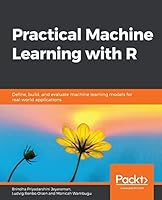# Practical Machine Learning with R## Book Description

Understand how works and get hands-on experience of using R to build that can solve various real-world problems

#### Key Features

• Gain a comprehensive overview of different machine learning techniques
• Explore various methods for selecting a particular algorithm
• Implement a machine learning project from problem definition through to the final model

#### Book Description

With huge amounts of data being generated every moment, businesses need applications that apply complex mathematical calculations to data repeatedly and at speed. With machine learning techniques and R, you can easily develop these kinds of applications in an efficient way.

Practical Machine Learning with R begins by helping you grasp the basics of machine learning methods, while also highlighting how and why they work. You will understand how to get these algorithms to work in practice, rather than focusing on mathematical derivations. As you progress from one chapter to another, you will gain hands-on experience of building a machine learning solution in R. Next, using R packages such as rpart, random forest, and multiple imputation by chained equations (MICE), you will learn to implement algorithms including neural net classifier, decision trees, and linear and non-linear regression. As you progress through the book, you'll delve into various machine learning techniques for both supervised and unsupervised learning approaches. In addition to this, you'll gain insights into partitioning the datasets and mechanisms to evaluate the results from each model and be able to compare them.

By the end of this book, you will have gained expertise in solving your problems, starting by forming a good problem statement, selecting the most appropriate model to solve your problem, and then ensuring that you do not overtrain it.

#### What you will learn

• Define a problem that can be solved by training a machine learning model
• Obtain, verify and clean data before transforming it into the correct format for use
• Perform exploratory analysis and extract features from data
• Build for neural net, linear and non-linear regression, classification, and clustering
• Evaluate the performance of a model with the right metrics
• Implement a classification problem using the neural net package
• Employ a decision tree using the random forest library

#### Who this book is for

If you are a data analyst, data scientist, or a business analyst who wants to understand the process of machine learning and apply it to a real dataset using R, this book is just what you need. Data scientists who use and want to implement their machine learning solutions using R will also find this book very useful. The book will also enable novice programmers to start their journey in data science. Basic knowledge of any is all you need to get started.

1. An Introduction to Machine Learning
2. Data Cleaning and Pre-Processing
3. Feature Engineering
4. Introduction to neuralnet and Evaluation Methods
5. Linear and Logistic Regression Models
6. Unsupervised Learning